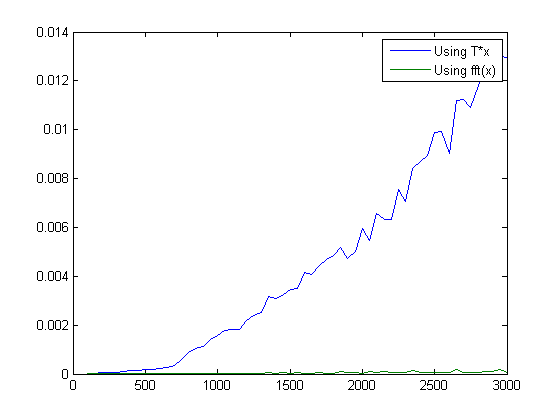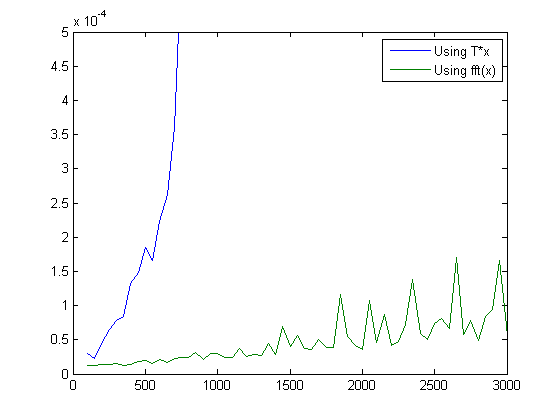# The DFT matrix and computation time

On my list of potential blog topics today I saw just this cryptic item labeled dftmtx. Hmm, the MATLAB dftmtx function. But have I written about this function before? I better double-check by searching the old blog postings:Oh, I forgot about Loren's post! She showed how the discrete Fourier transform, which MATLAB users normally compute by calling fft, can also be computed via a matrix multiply.

x = rand(1000,1);
X = fft(x);

T = dftmtx(1000);
X2 = T*x;

max(abs(X2(:) - X(:)))
ans =

3.9790e-13



The difference is just floating-point round-off error.

My old post talked about the value of having an independent computation method available when you are testing your algorithm.

So today let's do something a little different. Let's compare the performance of computing the discrete Fourier transform using the DFT matrix versus using the fast Fourier transform.

To help with the timing, I'm going to use a function I wrote called timeit that you can download from the MATLAB Central File Exchange.

clear
n = 100:50:3000;
for k = 1:length(n)
nk = n(k);
x = rand(nk,1);
T = dftmtx(nk);

f = @() T*x;
g = @() fft(x);

times_f(k) = timeit(f);
times_g(k) = timeit(g);
end

plot(n,times_f,n,times_g)
legend({'Using T*x', 'Using fft(x)'})That's a pretty dramatic difference. The blue curve, showing the computation time using T*x, is an n^2 curve. Compared to that, the green curve, showing the computation time using fft(x), is so low that you can hardly see it.

Let's expand the y axis.

ylim([0 0.0005])The lower green curve is an n*log(n) curve. The dramatic difference between that and the n^2 curve is why everyone got so excited when the fast Fourier transform algorithm was invented (or re-invented) a few decades ago.

Published with MATLAB® 7.14

|